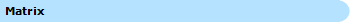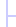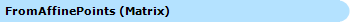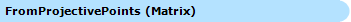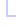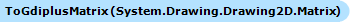This documentation is for the old version. Go to the latest Graphics Mill docs

# Matrix Class

This class represents a 3x3 matrix.

Namespace: Aurigma.GraphicsMill.Transforms
Assembly: Aurigma.GraphicsMill (in Aurigma.GraphicsMill.dll)

## Syntax

Visual Basic
```Public NotInheritable Class Matrix _
Inherits LockableObject```
C#
`public sealed class Matrix : LockableObject`

## Remarks

3x3 matrix is used to apply geometry transformation of the bitmap. Geometry transformation can be both affine (such as scale, skew or rotate) and projective (such as perspective correction).

You can create this class instance in two ways: either create new matrix and then fill it using FillFromProjectivePoints(PointF[], PointF[]) property, or generate it from points representing affine or projective transformation (see FromAffinePoints(PointF[], PointF[]) and FromProjectivePoints(PointF[], PointF[]) methods for more details).

This class also implements some matrix algebraic operations, such as matrix inversion (Invert() method), multiplication (Multiply(Matrix) method), determinant calculation (Determinant property). Also, you can apply affine operations at the matrix: rotate (Rotate(Single) and RotateAt(Single, PointF) methods), scale (Scale(Single, Single) method), and translate (Translate(Single, Single) method).

## Inheritance Hierarchy

System.Object
L Aurigma.GraphicsMill.LockableObject
L Aurigma.GraphicsMill.Transforms.Matrix

Static members of this type are safe for multi-threaded operations. Instance members of this type are safe for multi-threaded operations.

## Object Model